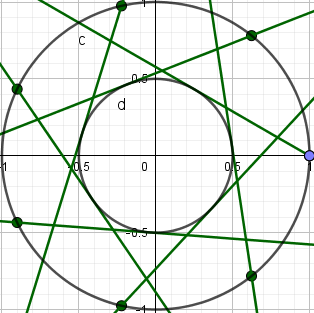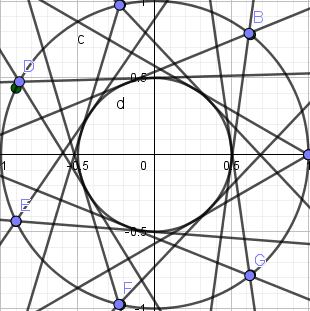# Sequence command doesn't consider all intersections and tangents

Clueid shared this problem 3 years ago
Not a Problem

Hello,

When putting Intersect() or Tangent() commands in Sequence() or Zip(), the command does not consider all possible tangents or intersections.sorry, I don't understand Your Question.

Verbinde ( Zip( { Tangente(P, d) } , P, l1))

----> {1

Another example:2

Have you tried Intersect(a,b,1) and Intersect(a,b,2)?1

Yes, but unfortunately, it does not work for tangents :(2

ein Versuch: l1: Liste von lines, c: circle

Verbinde(Zip({Schneide(c, g)}, g, l1))

W.F.12

Zip( { Schneide(c,t) },t,l2)

----->{ ---->}1

I don't want the second intersection of the tangents.

Given a list of points, I want to draw BOTH tangents from these list of points to the circle.I want this:From each point, TWO tangents should be drawn. Not one.1

sorry, I don't understand Your Question.

Verbinde ( Zip( { Tangente(P, d) } , P, l1))

----> {1

All right, thanks. This was what I wanted.2

You can also try

`{Intersect(f,g)}`1

Can you explain why the program doesn't from the beginning consider all possible intersections, please?